Next: Bibliography Up: Dissertation Siddhartha Dhar Previous: Appendix A

# Appendix B

From (4.118) and (4.119) we get(6.10)

After elimination of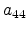, the system of equations (4.118) to (4.122) can be expressed in matrix form as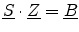(6.11)

where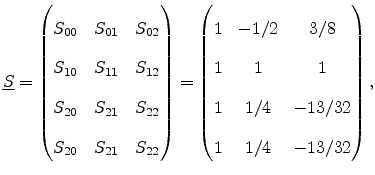(6.12)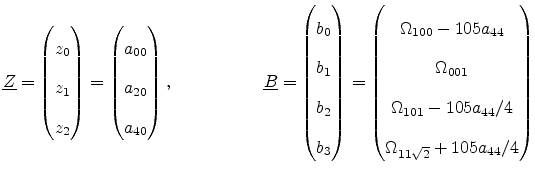(6.13)

To determine the four coefficients from the overdetermined system (6.11), we solve (4.118) to (4.120) exactly and minimize the error in  (4.121) and (4.122). Since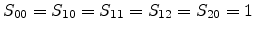, using the new variables the equations can be written as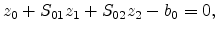(6.14)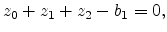(6.15)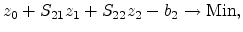(6.16)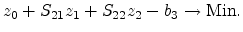(6.17)

Next, we eliminate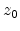by subtracting (6.15) from (6.14), (6.16), and (6.17).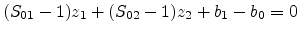(6.18)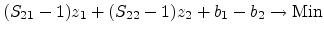(6.19)(6.20)

To eliminate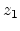, (6.18) is multiplied by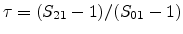to obtain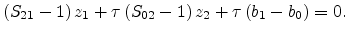(6.21)

Subtracting (6.21) from (6.19) and (6.20) gives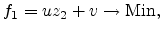(6.22)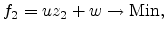(6.23)

with(6.24)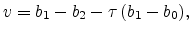(6.25)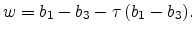(6.26)

The least square minimum can be obtained by setting the derivative to zero,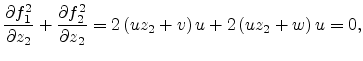(6.27)

which gives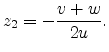(6.28)

With the definitions (6.24) to (6.26) and using (6.12) we get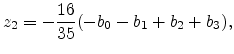(6.29)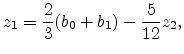(6.30)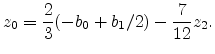(6.31)

Substituting back the value of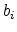's from (6.13) into (6.29) to (6.31) gives equations (4.123)-(4.126).Next: Bibliography Up: Dissertation Siddhartha Dhar Previous: Appendix A

S. Dhar: Analytical Mobility Modeling for Strained Silicon-Based Devices